Stress Analysis of GRP Pipes: According to ISO 14692-3

ISO 14692 is a development of UKOOA document. For definition of system load cases such as sustained, expansion and occasional load cases, the ASME B 31.3 methods are used and for system design parameters, flexibility factors, stress intensity factors (SIF) and pressure stress multipliers, the information presented in BS7159 are followed.

In order to maintain integrity of GRP process piping systems and pipelines, regarding following factors, the stress analysis should be performed to ensure that the system can sustain all stresses and deformations requirements.

• Pipe work flexibility

• Layout complexity

• Pipe supports

• Pipe work diameter

• Magnitude of temperature changes

• System criticality and failure risk assessment

Piping stress engineer should evaluate the total piping system in order to specify any need of flexibility analysis. For stress analysis, different loading conditions such as internal or external pressure, thermal, occasional and support loadings should be determined, and then the related stresses and loads should be evaluated and finally compared with the corresponding allowable stresses and loads respectively. In the following, the methods for evaluation of the mentioned loads, stresses, deflections and their allowable conditions are discussed. 

1-1- Qualified pressure

The qualified pressure, Pq is based on a design life of 20 years and determined by manufacturer based on the procedure described in sec 6.2.2 of ISO 14692-2.

1-2- Manufacturer’s pressure rating

The manufacturer’s pressure rating is defined as:(1)

Where:

= qualified pressure.

= load factor as defined in sec 7.6.2.2 of ISO 14692-3

= 0.5, for a component in a complex piping system, where significant non-pressure stresses can be produced.

= 0.9, if the component which is well supported and is a part of a long pipe run.

Note:    is not a fixed parameter and is strongly dependent on application and     of component. Manufacturer shall always provide the value of     . 

1-3- Factored qualified pressureThe factored qualified pressure is defined as a pressure which is used to determine a safe operating envelope of the GRP piping system, see section 3-3 of this article:(2)

Where:

= qualified pressure.

= temperature factor.

= chemical resistance factor.

= factor for cyclic services.

(1)

(2)

(3)

Notes:

• Partial factor     is determined according to annex D of ISO 14692-2; however if the operating temperature is less than or equal to 65 C, then      may be considered as 1.0.

• Partial factor      is determined in accordance with annex D of ISO 14692-2; However if the normal service fluid is water, then      may be considered as 1.0.

• If the predicted number of loading cycles is less than 7000, then      =1. If it exceeds 7000 over design life, then it may be defined as follows:(3)

Where:(4)

= maximum/minimum value of loads or stresses in the load or stress cycle respectively.

N = total number of cycle during services.1-4- External pressure/ vacuum

An external collapse pressure,     , of GRP pipes may be calculated by equation (5) provided that the pipe length is significantly larger than the diameter.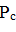(5)

Where:

= 1.5 for short term vacuum and 3.0 for long term vacuumThermally induced loads due to a maximum operating or ambient temperature range could be evaluated. The mean temperature change of a pipe wall may be calculated as:(6)

The induced loads due to a thermal expansion for any above ground piping system may be calculated; however, because of the low elasticity modulus values of GRP pipes, the expansion resulting from internal pressure can sometimes be equal in magnitude to thermal expansion. In the design of GRP piping systems, these expansions should be controlled by the following approaches: 

Where:

=Temperature difference between ambient temperature and the process design temperature, in degrees Celsius;

K = 0.85 for liquids and 0.8 for gases

• Controlling with anchors.

• Controlling with guides.

• Controlling with directional changes.

• Controlling with expansion loops.

• Controlling with bellows units.

2- Stresses

2-1- Stresses due to internal pressure

The hoop stress due to internal pressure may be calculated as: 

The axial stress due to internal pressure could be calculated as:The equivalent stress for fittings could be determined as:

(7)

(8)

(9)

2-2 Stresses due to pipe flexural bending moment

For gas services and small or medium- diameter pipes for liquid services, the generated local support stresses are considered insignificant comparing to the general axial stresses at mid-span. The magnitude of general axial stresses due to the pipe dead weight bending moment at mid-span may be calculated as: 

Where:(11)

(10)

(12)

(13)

(14)

The total general axial stress resulting from internal pressure and bending moment of self mass (or dead weight) at the bottom and top of the pipe is:

The deflection at mid-span due to dead weight may be determined as:

Where:

Ks = the support type factor; equals to 384 for single span beam (two supports); 925 for two span beam (three supports); 1920 for anchored beam (two fixed support at both ends)

A compressive longitudinal stress resulting from axial force may be checked against axial elastic bulking stress as:(15)

(16)

(17)

Where:

(18)

Note: The bulking stress to maximum compressive axial stress ratio shall be greater than 3.

2-3 Stresses due to pipe supports

For large diameter liquid filled pipes, with high D/t ratio, the local stresses at the support location become significant. By following calculations, the support local stresses may be determined provided that:

• The mass of GRP pipe is insignificant compared to the mass of liquid contents.

• The pipe material is isotropic

• The supports are flexible, for example they include an elastomeric pad. 

2-3-1 Local Axial stress in pipe at support location

At the highest point of a pipe cross section the axial stress may be calculated as:(19)

At the lowest point of the pipe cross section the axial stress may be calculated as: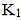Where:

Constant      is given in  for a range of saddle angle.

Note 1: In all cases                                      , K=5 for filament wound pipes.

Note 2: The above equation does not contain the effect of pressure. 

2-3-3 Local hoop stress in pipe at support location

At the nadir of saddle supports the hoop stress may be calculated as:(20)

(21)

(22)

Where:

Constant       is given in table  for a range of saddle angle, if the pipe and support are fixed together;      is one tenth of value given in .

b1 is the width of the saddle support.

At the saddle support horn the hoop stress may be calculated as: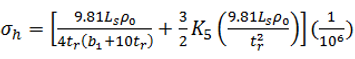(23)

(24)

Where:

Constant        is given in  for a range of saddle angle.

Note: The above equations do not include effect of internal pressure.

3- Allowable

3-1- Allowable pressure

The maximum allowable pressure for a component is:

Where:

= design pressure.

= qualified pressure

= safety part factor between the strength of the material and operating stress for three load cases: occasional, sustained including thermal loads and excluding thermal loads. This factor could be derived from table 2 and 3 of ISO 14692-3.(24)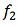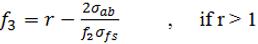Note: The magnitude of     depends on axial stress. 

The axial compressive system loads should not exceed             , which is calculated as follows:(26)

(27)

(28)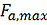Therefore, the equivalent Euler buckling stress is:

(29)

(30)

The qualified stresses for fitting could be calculated as:

The factored stress for a plain pipe could be determined as:

The factored stress for fitting is:

For filament wound GRP components, in ISO 14692,       is equivalent to hydrostatic design basis stress (HDBS) as defined in Section A302.3.2 of ASME B31.3 except that it has not been factored by      ,     , nor      .

The sum of all hoop stresses,              and all axial stresses,            , in any component in a GRP piping system due to pressure, mass, other sustained loads and occasional loads such as wind, blast or earthquake, shall not exceed the value defined by the factored long term design envelope, Figure 2 .or

Figure 2: Fully measured envelope

(31)

(32)

(33)

(34)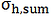The following equation may be used to determine the allowable hoop stress.

In ISO 14692, two design envelop have been defined based on available measure data as follows.

• Fully measured envelope

This envelope is generally available for a plain pipe, Figure 2. The short term envelope is derived according to the method presented in Annex C of ISO 14692-2:2002, then the idealized long term failure envelop is scaled according to         where:(35)

(36)

The non-factored long-term and factored long-term is defined according to part factor     and      .      .      respectively; therefore:• Simplified envelop

This method is more conservative than the fully measured envelop method and may be used for both plain pipe and other components, Figure 3.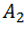(37)

Figure 3: Simplified envelopThe idealized long-term envelop could be determined as:

Where:

Therefore sum of all hoop stresses,            and all axial stresses,           , in any component in a GRP piping system shall not exceed the value defined by the factored long term design envelope as equation (41) and (43)(38)

(39)

(40)From equation (33) and (41):

(41)

From equation (40) and (42)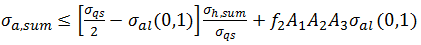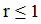(42)

(43)

(44)

A geometrical proof of equation (44) could be referred as follows, see figure 4.Figure 4: A geometrical proof for design envelop

By drawing line BS parallel to lin